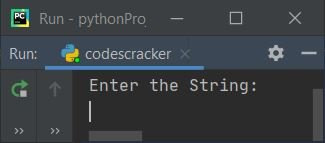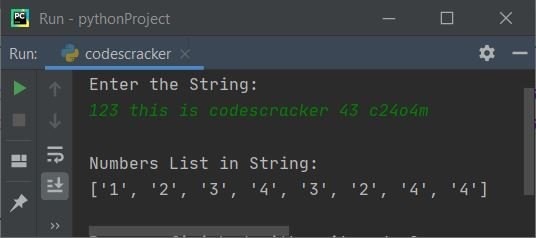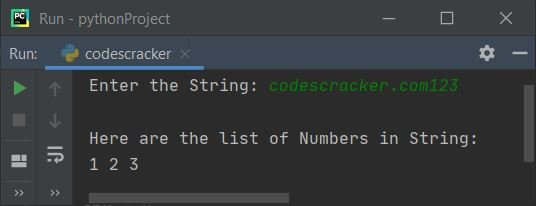# Python Program to Extract Numbers from a String

This article was created to cover some programs in Python that extract all numbers (0–9) from a given string at run-time. Here is a list of programs covered in this article:

• Extract numbers from a string using the "for" loop.
• Extract numbers from a string using the "for" loop and the "isdigit()" method.

## Extract numbers from a string using the for loop

The question is: write a Python program to extract numbers from a given string using a for loop. Here is its answer:

```print("Enter the String: ")
text = input()
textLen = len(text)
nums = []
for i in range(textLen):
if text[i]>='0' and text[i]<='9':
nums.append(text[i])
print("\nNumbers List in String:")
print(nums)```

Here is its sample run:Now supply the input "123, this is codescracker 43 c24o4m" as a string and press the ENTER key to extract all numbers from this string:## Extract numbers from a string using isdigit()

Now this program uses the "isdigit()" method to check whether the current character is a digit (0–9) or not, and then proceed further accordingly. The "end" in this program is used to skip the insertion of an automatic newline.

```print(end="Enter the String: ")
text = input()
textLen = len(text)
nums = []
chk = 0
for i in range(textLen):
if text[i].isdigit():
nums.append(text[i])
chk = 1
if chk==1:
print("\nHere is a list of Numbers in String: ")
numsLen = len(nums)
for i in range(numsLen):
print(end=nums[i] + " ")
else:
print("\nNumber is not available in the list!")```

Here is its sample run with user input, "codescracker.com123":Here is another sample run with user input, "codescracker" (string without number):Python Online Test

« Previous Program Next Program »